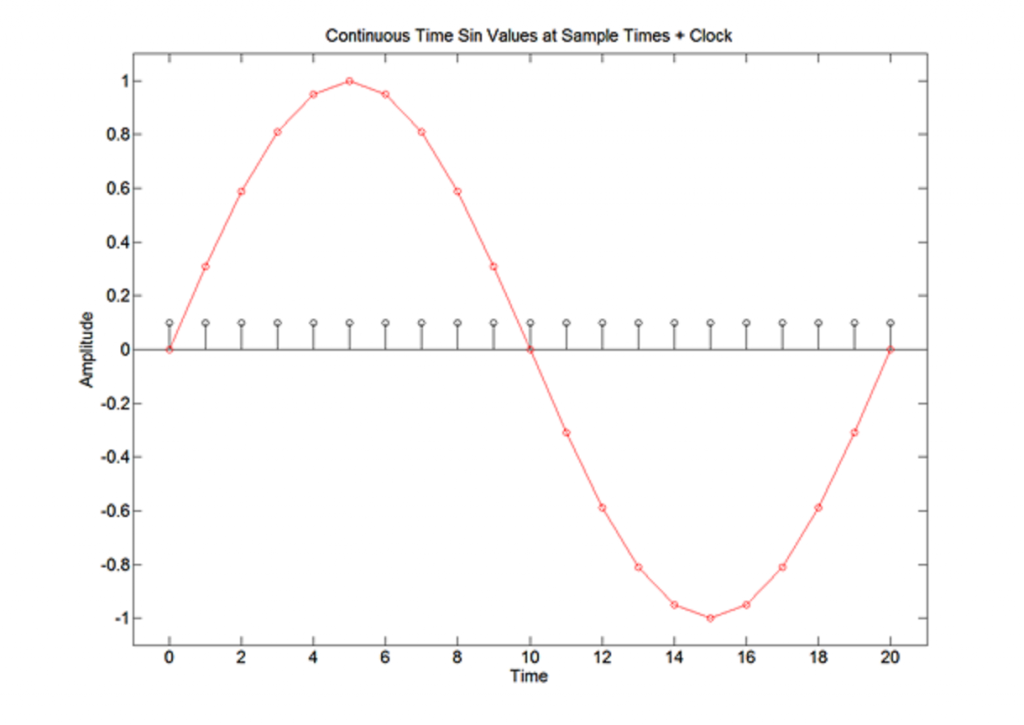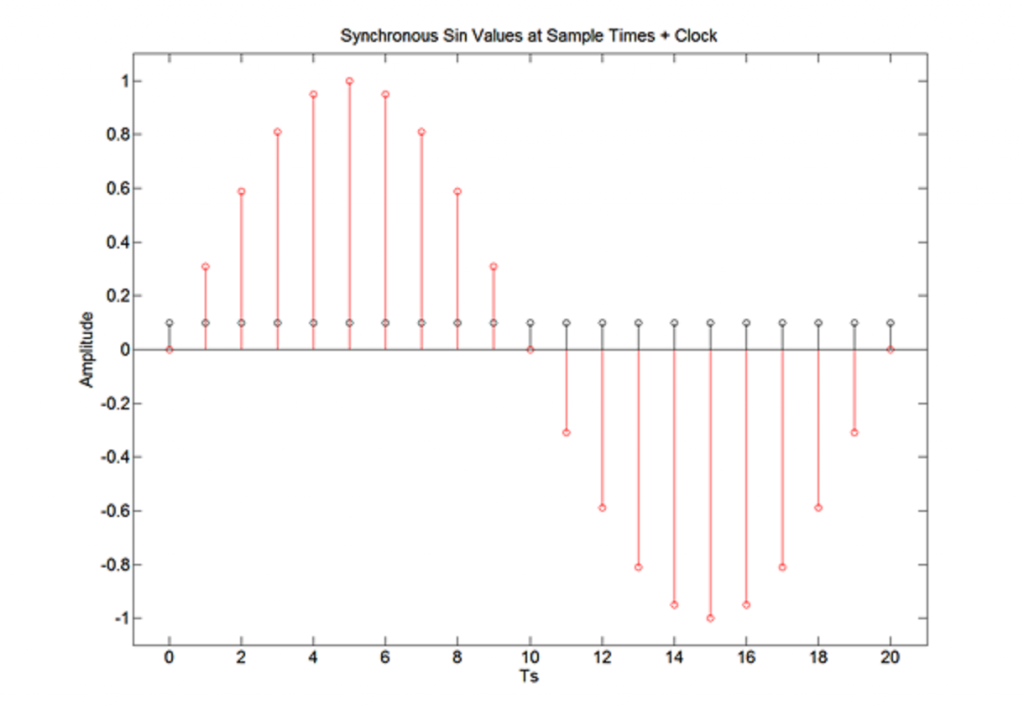### Overview

Analog input pins allow an application to examine the voltage levels present on a pin as an analog signal.   Analog signals are used to measure signals that are continuous in nature.  Examples of such a signals would be a sine wave or an EKG.  The frequency and magnitude of  an analog signal has meaning.   When we examine an analog signal we are interested in the the continuous nature of the signal, not just a if the signal exceeds a specified threshold.  This differs from how a digital signal is interpreted.  For a digital signal, we only care if the voltage level is greater than VIHMIN or less than VILMAX.  As long as we do not cross one of these thresholds, the exact magnitude of the input voltage at any given time is somewhat irrelevant.

### Quantization

In order to analyze an analog signal, the application must sample the analog signal and reconstruct it in the digital domain.  A microprocessor samples an analog signal using an analog to digital converter (ADC).  The ADC takes the magnitude of the voltage and converts it to a binary value representing the magnitude.

One of the most important characteristics of an ADC is how many bits the ADC uses to represent the magnitude of a signal.  The number of bits in a sample is often times referred to as the number of bits of precision.  The more bits of precision, the more precisely we can measure an analog signal.  In order to explain why this is true, we will use a simple example.

Let’s assume that we have a signal that varies between 3.3V and 0.0V.  Let’s also assume that we have an ADC has 3 bits of precision.  With 3 bits of precision, the ADC can return one of eight unique values.  Based on having eight possible values, we can determine the resolution of the ADC to be 3.3V/8 = 0.4125.

If the ADC returned a value of 0002 what voltage would this value represent? The answer is 0002 could represent any value from 0.0V to 0.4124V.     A value of 0012 represents any value from 0.4125V to 0.824V.

If we were to increase the number of bits of precision to be 10 bits, the number of possible values returned by the ADC is now 1024 distinct values.  The resolution of the ADC is now 3.3/1024 = 0.0032.   An ADC value of 00000000002 represents a magnitude of 0.0V to 0.0032V.  It should be fairly obvious that increasing the number of bits of precision allows us to more accurately reconstruct a signal.

### Sampling Rate

An application can reconstruct an analog signal in the digital domain by examining how the magnitude of the signal changes over a given period of time.  The diagram below shows a continuous sine wave and the locations where a sample will be taken.We can use these sample locations to determine the magnitudes returned to the microprocessor.The graph above helps to visualize the original sine wave.  If we increase rate at which we sample, we can more accurately represent the sine wave.  In an ideal situation, an application would sample at an infinitely fast rate.  While this is ideal, it is not possible.  Our microprocessors have a limit to how fast they can operate.  There are also other operations that an application must carry out that will decease how frequently we can dedicate the CPU to sampling an analog signal.   So instead of sampling at an infinitely high rate, we will sample at more than twice the highest frequency of the signal that you want to measure.  This sampling rate is called the Nyquist sampling rate.  By sampling at a minimum rate of twice the maximum frequency, we help to ensure that we do not have aliasing of our signal and can accurately reconstruct the signal.